Courses

# Torsion of Shafts Civil Engineering (CE) Notes | EduRev

## Civil Engineering (CE) : Torsion of Shafts Civil Engineering (CE) Notes | EduRev

The document Torsion of Shafts Civil Engineering (CE) Notes | EduRev is a part of the Civil Engineering (CE) Course Civil Engineering SSC JE (Technical).
All you need of Civil Engineering (CE) at this link: Civil Engineering (CE)

Chapter 8

Torsion of Shafts

TORSION OF CIR CULAR  SHAFTS
Theory of Pure Torsion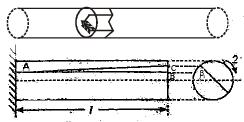Torsional Moment of Resistance: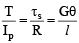ts= Shear intensity at the surface of the shaft
R = Radius of shaft
G = Modulus of rigidity of shaft material
l = Length of shaft
θ = Angular movement due to strain in length of the shaft
T = total moment of resistance offered by the cross-section of the shaft
I|p= Polar moment of Inertia of the section of the shaft

Assumptions in the theory of pure torsion: 

• The material of the shaft is uniform throughout. 
• Twist along the shaft is uniform. 
• Shaft is of uniform circular section throughout, which may be hollow or solid. 
• Cross section of the shaft, which are plane before  twist remain plane after twist. 
• All radii which are straight  before twist remain straight after twist: Polar modulus:

Polar modulus =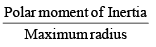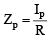• The greatest twisting moment which a given shaft section can resist = Max. permissible shear stress × Polar Modulus

Þ T = δs Zp 

• For solid shaft,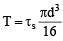for hollow shaft,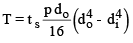Where, do = outer diameter
di = inner diameter

Torsional rigidity: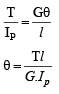Where, G = rigidity modulus
Ip = Polar moment of Inertia

The quantity GIp is called torsional rigidity. It is the torque required to produce a  twist of 1 radian per unit length of the shaft.
Power Transmitted by a shaft: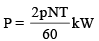= Torque × angle turned per second Where,
P = Power transmitted (kW)

N = rotation per minute (rpm)
T = mean torque (kNm)

SHAFTS IN SERIES AND SHAFTS IN PARALLEL
(a) shafts in series: 

• Torque T will be same for both the shafts. 
• The  twists q1 and q 2 will be different for both the shafts.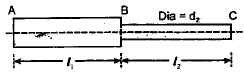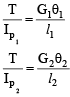Where, T = Torque G1, G2 = Modulus of rigidity for shafts 1& 2 l1, l2 = length of shaft 1&2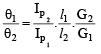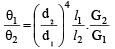if l1 = l2 G1 = G2 them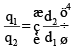Where, q1, q2 = angle of twist Ip1, Ip2 = polar moments of inertia

(b) Shafts in parallel: 

• In this case applied torque T is distributed to two shafts.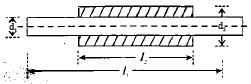T = T1 + T2
 The angle of twist will be same for each shaft,
q1 = q2=q 

T = T1 + T2 =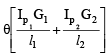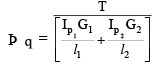COMPARISON BETWEEN SOLID AND HOLLOW SHAFTS
Let hollow shaft and solid shafts have same material and length.
D0 = external diameter of hollow shaft
Di = nD0 = Internal diameter of hollow shaft
D = Diameter of the solid shaft

Case (i): When the hollow and solid  shafts have the same torsional strength.

•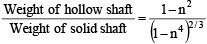• In this case polar modulus section of two shafts would be equal.
•
• USE: % Saving  in weight can be calculated for same torsional strength.

Case (ii): When the hollow and solid shafts are of equal weights. 

• In this case torsional strength is compared.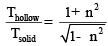• USE: ratio of strength for same weight can be calculated.

Case (iii) : When the diameter of solid shaft is equal  to the external diameter of the hollow shaft.

•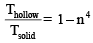SHEAR AND TORSIONAL RESILIENCE
Shear resilience: Let t = shear stress intensity at faces of a square block 

• Strain energy stored per unit volume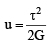(uniform through the section)

Where G = rigidity modulus.

Torsional resilience: 

• In this case shear stress due to torsion varies uniformly form zero at the axis to the maximum value ζs at the surface. 
• Strain energy stored, per unit volume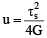• for hollow shaft,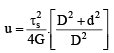Where, D = outer diameter of hollow shaft
d = internal diameter of hollow shaft

Offer running on EduRev: Apply code STAYHOME200 to get INR 200 off on our premium plan EduRev Infinity!

## Civil Engineering SSC JE (Technical)

113 docs|50 tests

,

,

,

,

,

,

,

,

,

,

,

,

,

,

,

,

,

,

,

,

,

;